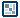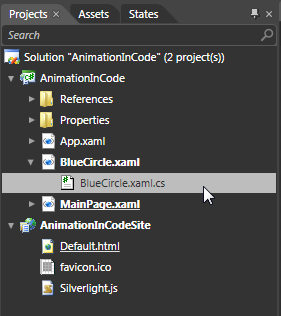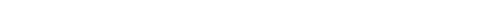## Question of the WeekCreating Killer Animations in Code - Page 3        by kirupa  |  17 October 2009 In the previous page, you converted your circle into a UserControl and made sure its parent layout container was a Canvas. All of that is just the preparation for what we are about to do here. Read on! Adding the Code to Make the Circle Move The next step is to add some code to make your circle actually move. From your Projects panel, expand BlueCircle.xaml by clicking on the tiny arrow found left of it and open BlueCircle.xaml.cs that appears nested directly under it by double-clicking on it:[ open BlueCircle.xaml.cs ] When you double-click the BlueCircle.xaml.cs file, Blend will open that file in its code-editor where you can write and edit code using a lot of the cool functionality that you would expect from a code-editing environment. If I ignore the using statements and namespace declaration, the code that you see currently will look as follows: public partial class BlueCircle : UserControl { public BlueCircle() { // Required to initialize variables InitializeComponent(); } } What you are going to do next is copy and paste some code. Copy the following code and paste it directly over the entire chunk of code defined in the public BlueCircle() block: private static Random randomMain = new Random();   private double angle; private double speed;   private double xPos; private double yPos;   public BlueCircle() { // Required to initialize variables InitializeComponent();   this.Loaded += new RoutedEventHandler(CircleLoaded); }   void CircleLoaded(object sender, RoutedEventArgs e) { xPos = Canvas.GetLeft(this); yPos = Canvas.GetTop(this);   speed = .01 + randomMain.NextDouble();   if (DesignerProperties.GetIsInDesignMode(this) == false) { CompositionTarget.Rendering += new EventHandler(AnimateCircle); } }   void AnimateCircle(object sender, EventArgs e) { angle += speed/10;   Canvas.SetTop(this, yPos + 50 * Math.Sin(angle)); Canvas.SetLeft(this, xPos + 50 * Math.Cos(angle));   if (angle >= 2 * Math.PI) { angle = 0; } } After you have pasted that code, there is one more copy/paste action that you need to do. In the list of using statements you see at the top of your code file, paste the following directly below using System.Windows.Shapes: using System.ComponentModel; Once your code has been pasted in, your BlueCircle.xaml.cs file will basically look as follows: using System; using System.Windows; using System.Windows.Controls; using System.Windows.Documents; using System.Windows.Ink; using System.Windows.Input; using System.Windows.Media; using System.Windows.Media.Animation; using System.Windows.Shapes; using System.ComponentModel;   namespace { public partial class BlueCircle : UserControl { private static Random randomMain = new Random();   private double angle; private double speed;   private double xPos; private double yPos;   public BlueCircle() { // Required to initialize variables InitializeComponent();   this.Loaded += new RoutedEventHandler(CircleLoaded); }   void CircleLoaded(object sender, RoutedEventArgs e) { xPos = Canvas.GetLeft(this); yPos = Canvas.GetTop(this);   speed = .01 + randomMain.NextDouble();   if (DesignerProperties.GetIsInDesignMode(this) == false) { CompositionTarget.Rendering += new EventHandler(AnimateCircle); } }   void AnimateCircle(object sender, EventArgs e) { angle += speed/10;   Canvas.SetTop(this, yPos + 50 * Math.Sin(angle)); Canvas.SetLeft(this, xPos + 50 * Math.Cos(angle));   if (angle >= 2 * Math.PI) { angle = 0; } } } } It is time to test it out and see how it works. Press F5 (or Project | Run) to load your browser and load your application. If everything went well, you will see your circle slowly moving! Now that you have a working project, let's next look at why the code works the way it does in greater detail on the next page. Onwards to the next page!